### Vectors and their Operations: Vector components

If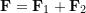, it implies that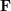has two components along the directions or lines of action of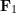and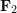(Fig. 2.13). We can consider decomposing the vectorinto two vector componentsand. Given a set of two directions, a unique component of a vector along each direction can be found by decomposing the vectors. Vector decomposition ofcan be regarded as the reverse of the vector addition, which means finding vectors with known directions (but unknown magnitude) such that their addition equals. Decomposing a vector can be performed using the parallelogram rule and trigonometry laws.

Vector decomposition produces components of vectors that are parallel with particular axes. The following interactive example shows the process of vector decomposition.

### EXAMPLE 2.3.1

The vectorof magnitude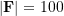is to be resolved into two components along the linesand. Determine the angleif the component ofalong the linehas a magnitude of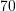, i.e.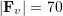.

#### SOLUTION

Use the parallelogram rule on the given directions of the component vectors.because of the parallel sides.

From Sine Law,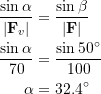#### EXAMPLE 2.3.2

Determine the resultant vector,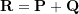.

#### SOLUTION

Two methods:

Method 1

From geometry: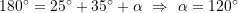.

From Cosine Law: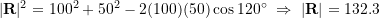.

From Sine Law: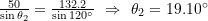.

Answer: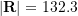,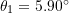parallelogram method, the use of Sine and Cosine laws is cumbersome. To add vectors efficiently systematically, vectors can be decomposed (resolved) onto a set of common directions and the resulting parallel vector components can be simply added.

Method 2

By decomposing the vectors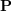and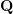on the two perpendicular lines (axes) denoted as x and y.

Components ofare as,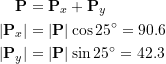Components of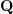are as,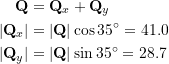Solving for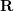: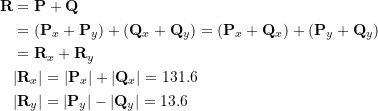Therefore: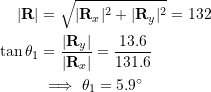Answer: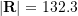,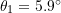In the above example, the vectors are resolved into their components on the given axes and the parallel components of the vectors are added. Then, adding the resultant vectors on the axes gives the final resultant vector. This approach is a systematic approach for adding vectors.